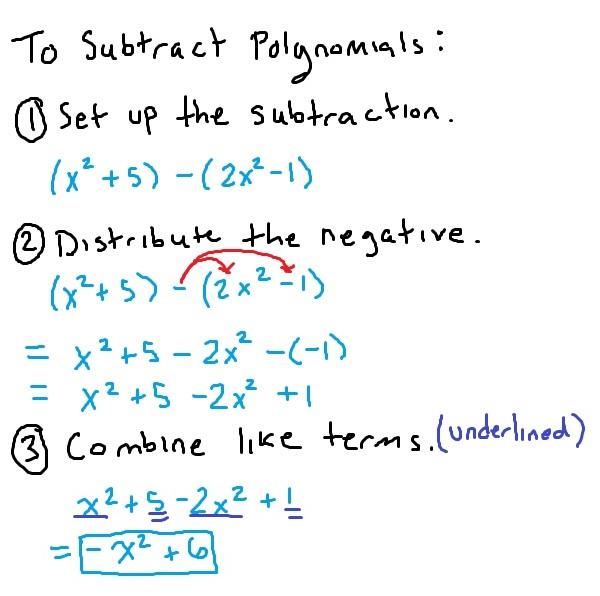As you will see below, in order to add or subtract polynomials, you really just need to pay attention to signs and combine like terms. This is especially important with subtraction, as you will be distributing a negative sign.

There are only really two steps required for adding two polynomials. For example, consider adding$x^2 + 4x + 1$ and$4x^2 - 2$. In the picture, like terms are underlined the same number of times.For practice, let’s apply these steps to a couple more problems.

### Example

Find the sum:$-3x^4 + 5x^3 - 7$ and$-8x^4 - 10x^3 + 3x + 2$

Remember here that “find the sum” is another way of saying “add”. So, we will first set up the addition and then combine like terms. Just as in the picture, like terms are underlined.$(-3x^4 + 5x^3 - 7) + (-8x^4 - 10x^3 + 3x + 2)$$= -\underline{3x^4} + \underline{\underline{5x^3}} - \underline{\underline{\underline{7}}} -\underline{8x^4} -\underline{\underline{ 10x^3}} + 3x + \underline{\underline{\underline{2}}}$$= \boxed{-11x^4 -5x^3 + 3x -5}$

In some problems, you may find that the set up is already done. In these cases, you need only worry about combining like terms.

### Example

Simplify:$(14y^3 + 2y^2) + (3y^3 + 7y^2)$

Although the instructions say simplify, you can see that this is actually a polynomial addition problem. Therefore, we can find the answer with the following steps.$(14y^3 + 2y^2) + (3y^3 + 7y^2)$$=\underline{14y^3} + \underline{\underline{2y^2}} + \underline{3y^3} + \underline{\underline{7y^2}}$$=\boxed{17y^3 + 9y^2}$

## Subtracting polynomials

When subtracting two polynomials, there is one extra step we must apply before combining like terms: distribute the negative. As an example, consider$(x^2 + 5) - (2x^2 -1)$.Notice that when you distribute the negative, you distribute it to every term in the parentheses. Pay close attention to this in the next couple of examples.

### Example

Find the difference:$(-4x^3 + x^2 - 1) - (-2x^3 + 3x^2 + 5)$

Just as “find the sum” means to add, “find the difference” means to subtract. Therefore, we will set up the subtraction, distribute the negative, and then collect like terms to find the answer.$(-4x^3 + x^2 - 1) - (-2x^3 + 3x^2 + 5)$$= -\underline{4x^3} + \underline{\underline{x^2}} - 1 + \underline{2x^3} - \underline{\underline{3x^2}} - 5$$= \boxed{-2x^3 -2x^2 -6}$

As you can see, the main idea is to keep track of the signs. Also note, that just as with addition, instructions may just say “simplify”. Even so, you will still distribute the negative before combining like terms.

### Example

Simplify:$(12x^5 -x) - (18x^5 + 4x^2 + x + 1)$$=\underline{12x^5} -\underline{\underline{x}} - \underline{18x^5} - 4x^2 - \underline{\underline{x}} - 1$$=\boxed{-6x^5 - 4x^2 - 2x - 1}$

## Summary

Now that you have studied how to add and subtract polynomials, let’s review the overall steps.

1. Set up the addition or the subtraction.
2. Distribute the negative (if subtracting).
3. Combine like terms / simplify.

You will find later that these skills are important when working with rational expressions, long division of polynomials, and even when working with functions!